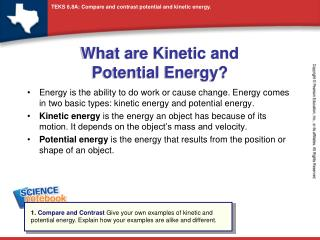DownloadDownload PresentationWhat are Kinetic and Potential Energy?

# What are Kinetic and Potential Energy?

Télécharger la présentation## What are Kinetic and Potential Energy?

- - - - - - - - - - - - - - - - - - - - - - - - - - - E N D - - - - - - - - - - - - - - - - - - - - - - - - - - -
##### Presentation Transcript

1. What are Kinetic and Potential Energy? • Energy is the ability to do work or cause change. Energy comes in two basic types: kinetic energy and potential energy. • Kinetic energy is the energy an object has because of its motion. It depends on the object’s mass and velocity. • Potential energy is the energy that results from the position or shape of an object. 1. Compare and ContrastGive your own examples of kinetic and potential energy. Explain how your examples are alike and different.

2. What Are Types ofPotential Energy? • Gravitational potential energy is potential energy related to an object’s height or weight. • Elastic potential energy is the energy associated with objects that can be compressed or stretched.

3. How are Potential and Kinetic Energy Related? • When an object moves, potential energy transforms into kinetic energy. An object at rest has potential energy but no kinetic energy. 2. ExplainHow do the potential energy and kinetic energy of a ball change as the ball rolls down a hill?